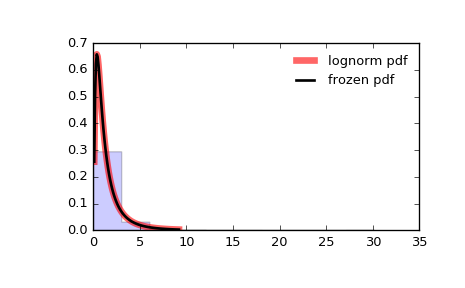# scipy.stats.lognorm¶

scipy.stats.lognorm = <scipy.stats._continuous_distns.lognorm_gen object at 0x2b153cf044d0>[source]

A lognormal continuous random variable.

As an instance of the rv_continuous class, lognorm object inherits from it a collection of generic methods (see below for the full list), and completes them with details specific for this particular distribution.

Notes

The probability density function for lognorm is:

lognorm.pdf(x, s) = 1 / (s*x*sqrt(2*pi)) * exp(-1/2*(log(x)/s)**2)


for x > 0, s > 0.

lognorm takes s as a shape parameter.

The probability density above is defined in the “standardized” form. To shift and/or scale the distribution use the loc and scale parameters. Specifically, lognorm.pdf(x, s, loc, scale) is identically equivalent to lognorm.pdf(y, s) / scale with y = (x - loc) / scale.

A common parametrization for a lognormal random variable Y is in terms of the mean, mu, and standard deviation, sigma, of the unique normally distributed random variable X such that exp(X) = Y. This parametrization corresponds to setting s = sigma and scale = exp(mu).

Examples

>>> from scipy.stats import lognorm
>>> import matplotlib.pyplot as plt
>>> fig, ax = plt.subplots(1, 1)


Calculate a few first moments:

>>> s = 0.954
>>> mean, var, skew, kurt = lognorm.stats(s, moments='mvsk')


Display the probability density function (pdf):

>>> x = np.linspace(lognorm.ppf(0.01, s),
...                 lognorm.ppf(0.99, s), 100)
>>> ax.plot(x, lognorm.pdf(x, s),
...        'r-', lw=5, alpha=0.6, label='lognorm pdf')


Alternatively, the distribution object can be called (as a function) to fix the shape, location and scale parameters. This returns a “frozen” RV object holding the given parameters fixed.

Freeze the distribution and display the frozen pdf:

>>> rv = lognorm(s)
>>> ax.plot(x, rv.pdf(x), 'k-', lw=2, label='frozen pdf')


Check accuracy of cdf and ppf:

>>> vals = lognorm.ppf([0.001, 0.5, 0.999], s)
>>> np.allclose([0.001, 0.5, 0.999], lognorm.cdf(vals, s))
True


Generate random numbers:

>>> r = lognorm.rvs(s, size=1000)


And compare the histogram:

>>> ax.hist(r, normed=True, histtype='stepfilled', alpha=0.2)
>>> ax.legend(loc='best', frameon=False)
>>> plt.show()Methods

 rvs(s, loc=0, scale=1, size=1, random_state=None) Random variates. pdf(x, s, loc=0, scale=1) Probability density function. logpdf(x, s, loc=0, scale=1) Log of the probability density function. cdf(x, s, loc=0, scale=1) Cumulative density function. logcdf(x, s, loc=0, scale=1) Log of the cumulative density function. sf(x, s, loc=0, scale=1) Survival function (also defined as 1 - cdf, but sf is sometimes more accurate). logsf(x, s, loc=0, scale=1) Log of the survival function. ppf(q, s, loc=0, scale=1) Percent point function (inverse of cdf — percentiles). isf(q, s, loc=0, scale=1) Inverse survival function (inverse of sf). moment(n, s, loc=0, scale=1) Non-central moment of order n stats(s, loc=0, scale=1, moments='mv') Mean(‘m’), variance(‘v’), skew(‘s’), and/or kurtosis(‘k’). entropy(s, loc=0, scale=1) (Differential) entropy of the RV. fit(data, s, loc=0, scale=1) Parameter estimates for generic data. expect(func, args=(s,), loc=0, scale=1, lb=None, ub=None, conditional=False, **kwds) Expected value of a function (of one argument) with respect to the distribution. median(s, loc=0, scale=1) Median of the distribution. mean(s, loc=0, scale=1) Mean of the distribution. var(s, loc=0, scale=1) Variance of the distribution. std(s, loc=0, scale=1) Standard deviation of the distribution. interval(alpha, s, loc=0, scale=1) Endpoints of the range that contains alpha percent of the distribution

#### Previous topic

scipy.stats.loglaplace

#### Next topic

scipy.stats.lomax